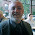### Algorithm Bleg

I want to position agents on a grid consisting of X*Y cells. I want to plop them around randomly. But I don't want to put them in a cell containing another agent. Right now, I have the following function:
```     def position_agent(self, agent, x=RANDOM, y=RANDOM):        """         Position an agent on the grid.        If x or y are positive, they are used, but if negative,         we get a random position.        Ensure this random position is not occupied (in Grid).        """        if x == RANDOM or y == RANDOM:            got_cell = False            while not got_cell and self.exists_empty_cells():                x = random.randint(0, self.width - 1)                y = random.randint(0, self.height - 1)                if self.is_cell_empty(x, y):                     got_cell = True            if not got_cell:                logging.error("Grid full; "                              + agent.name + " not added.")                return            self.grid[y][x] = agent        self.filled_cells += 1 ```

This function works, but the problem with it is the maximum potential run-time is essentially unbounded: As the grid gets filled up, this function simply relies on random.randint() to eventually hit upon an empty cell! One potential solution is to check how full the grid is, and once it gets past a certain percentage, just place each successive agent in one of the empty places. But that strikes me as kludgy. Any ideas on how to do this better?

1.Not a computer guy, but random thought --

1) make your x,y cells into a dictionary or database or something (key-value pairs); keys = 1 to x*y
2) as you randomly pick out of the dictionary, remove them from the dictionary. (by choosing between 1 and (X*y - times you've been through the loop))

Or something like that...

2.You can create a list of all possible x,y co-ordinate pairs, then randomly pick pairs from the list, removing them as you do so. Then to select n pairs will need exactly n attempts, and you are guaranteed no duplicates.

3.Sorry, made that too complicated. Just make a list, and use the indexes of the list. Remove entries from the list as you use them up. You don't need to get as complex as a dictionary or database...

4.Sorry, my last comment was a bit glib as I did not consider the cost of the data structure. Finding and removing the nth element from either an array or linked list will require O(n) time, resulting in an undesirable overall O(n^2) run-time. More detail required, but I am going back to bed.

1.Yes, but that O(n^2) is much better than an unbounded run-time! And it is only done once, as agents are setup.

2.Why not shuffle the list (O(n)) at the start and then pick the first element?

5.Looks like what you want is a 'shuffle algorithm' such as Fisher–Yates shuffle - then you take a list of your co-ordinates, shuffle them, and pick the first n.

1.Exactly. N agents, pick the first N elements from a shuffled list.

(This could be a problem if the number of elements is >> N. But in that case then in fact the chance of collisions will be slim and you can just generate random numbers. )

6.One idea: if you want to place k agents, take the first k pairs in the output of numpy.random.permutation([(x,y) for x in range(X) for y in range(Y)]).

7.The shuffled list of coordinates is a good solution if you don't need to move the agents around and it fits in memory.

If you do need to move agents around efficiently and/or you want to get fancy for fun, you could use a data structure called a "quadtree". Recursively decompose the 2D space into four squares. For each square (at each level of decomposition) store how much room there is remaining in its enclosed space.

When you need to find a place to put an agent, start at the top and randomly choose one of the 1-4 squares with room remaining, then recurse until you get to a final cell with room. When you add the agent, recurse back up to decrement the remaining room at each level. Both operations are O(log n).

8.Create an index of empty cells and remove items from that as necessary.

9.Create an array that stores available cells as a pair of x- and y-coordinates and used your random number generator to select a coordinate pair in that array. Remove said item after selection.

10.Thanks for the great suggestions! The newest post shows what I did, based on these.

11.You could always traverse the grid looking for an empty space , but just start the search from a random place and go back to 0,0 when you reach the end of the grid without having found an empty slot.

12.Nice rob!